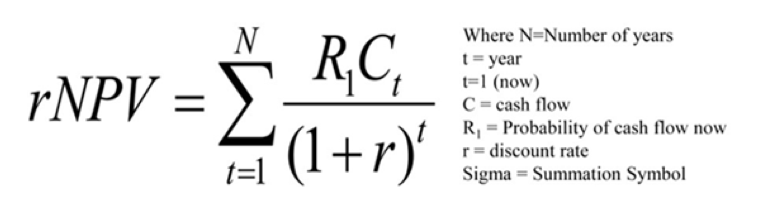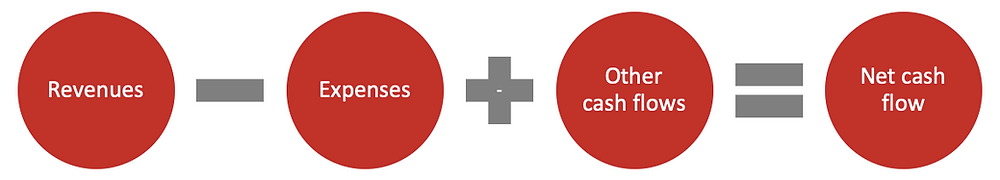Search
•Friday Capital

# Valuing life sciences companies using the rNPV methodologyThe predominant tool used to value drug development projects is Risk Adjusted NPV (rNPV) as discussed in Part 2 of this series.

The formula for rNPV is expressed as follows:But this can be more simply expressed in 3 main steps to follow in order to calculate rNPV (as shown in the following diagram).Let’s run through each of the 3 steps in a little more detail.

1. Estimate Net Cash Flows

Net cash flows are calculated as revenues less expenses plus other cash flows.There are two approaches used to estimate revenues from drug sales: (1) top-down: forecast the size of the market and the market share likely to be attained by the drug candidate, or (2) bottom-up: focus on the number of patients and drug price.

Expenses include R&D costs, drug production costs (typically 20% of sales) and SG&A expenses (usually 35% of sales).

Other cash flows consist of items such as changes in working capital, cash taxes etc.

2. Adjust the net cash flows by risk weightings

The cash flows of future phases are adjusted by the probability of successfully progressing the program to each of those respective phases.

After averaging the findings of 16 studies, we have come up with the following table to provide probability adjustment amounts.For example, if a product is currently at the preclinical phase, there is a 19% chance of it reaching the market and all cash flows relating to drug sales would need to be weighted by 19%. Furthermore, R&D costs from each subsequent phase would also need to be weighted (in our example, phase I costs would be weighted by 76%).

3. Divide the risk adjusted cash flows by an annual discount rate (to get the value of the drug asset in today’s terms)

The discount rate reflects the general risk for drug development companies, and it factors in management, financing, manufacturing, and IP risks. Note that the product development risk is not included as a component of the discount rate (as it has already been used to adjust net cash flows).

The actual discount rate used will depend on the maturity stage of the bioscience company: (1) early stage 18-24%; (2) mid stage 16-20%; or (3) late stage 10-16%. 

To sum up, in order to calculate the value of a drug program according to rNPV, we need to adjust the estimated net cash flows of the drug program by probability weightings and then apply an annual discount rate to these adjusted cash flows.